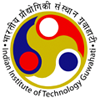•Department of Electronics and Electrical Engineering
Indian Institute of Technology GuwahatiDepartment of Electronics
and Electrical Engineering

### VLSI DSP

Course Contents:

Introduction to DSP systems: Representation of DSP algorithms; Iteration Bound: Definition, Examples, Algorithms for computing Iteration bound; Pipelining and Parallel Processing: Definitions, Pipelining and parallel processing of FIR filters, Pipelining and parallel processing for low power; Retiming: Definitions and Properties, Solving system of Inequalities, Retiming techniques; Unfolding: Definition, An algorithm for unfolding, Applications of unfolding; Folding: Definition, Folding transformations, Register minimization techniques, Register minimization in folded architectures; Systolic Architecture Design: Introduction, Systolic array design methodology, FIR systolic arrays, Selection of scheduling vector, Matrix-Matrix multiplication and 2D systolic array design; CORDIC based Implementations: Architecture, Implementation of FIR filter and FFT algorithm; Bit-Level arithmetic architectures: Parallel multipliers, Bit-serial multipliers, Bit-Serial FIR filter design and Implementation; Redundant arithmetic: Redundant number representation, Carry-free radix-2 addition and subtraction, radix-2 hybrid redundant multiplication architectures; Low-power design: Theoretical background, Scaling versus power consumption, Power analysis, Power reduction techniques, Power estimation approaches.

Texts / References:

1. U. Meyer-Baese, DSP with FPGA, Springer, 2004.
2. K. K. Parhi, VLSI DSP Systems, Wiley, 2003.
3. R.G. Lyons, Understanding Digital Signal Processing, Pearson Education, 2004.